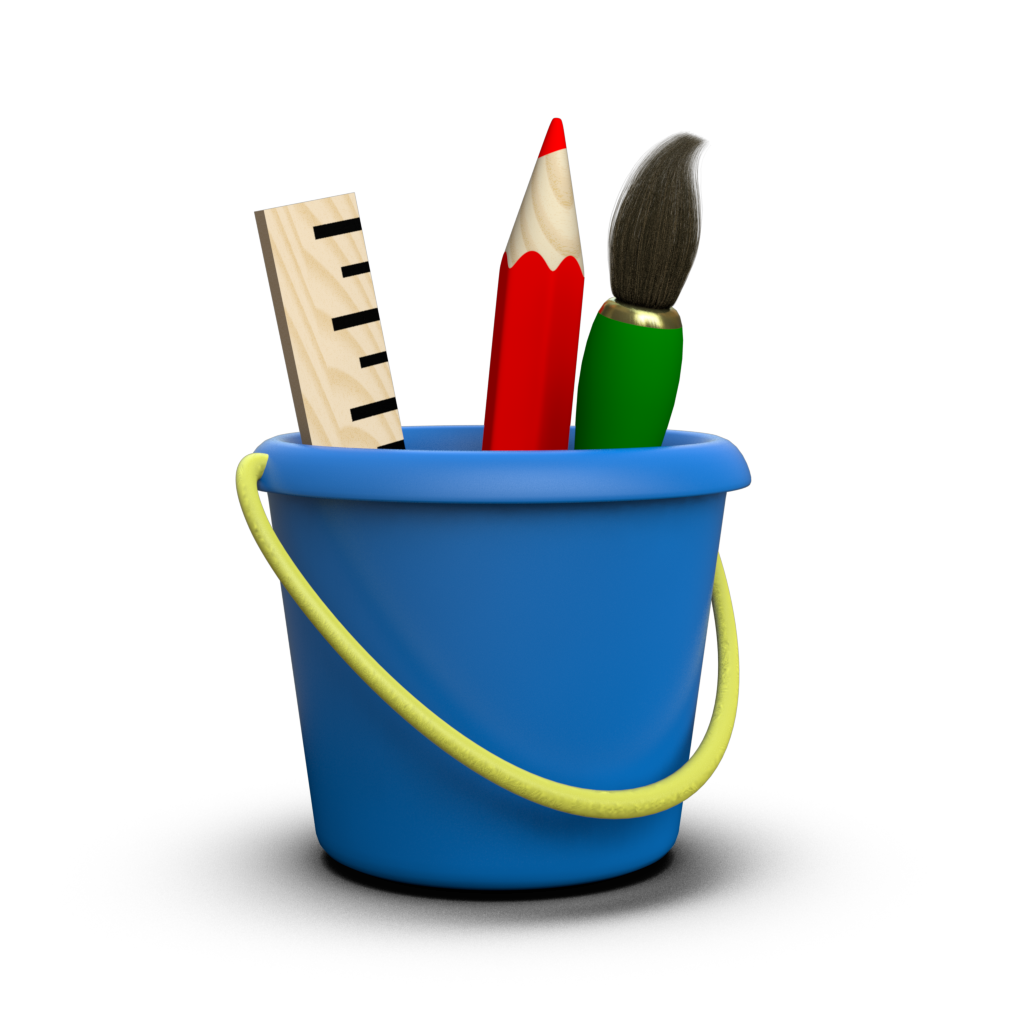# Markers¶

In Toyplot, markers are used to show the individual datums in scatterplots:

:

import numpy
import toyplot

import logging
logging.basicConfig()

:

y = numpy.linspace(0, 1, 20) ** 2
toyplot.scatterplot(y, width=300);


Markers can also be added to regular plots to highlight the datums (they are turned-off by default):

:

canvas = toyplot.Canvas(600, 300)
canvas.cartesian(grid=(1, 2, 0)).plot(y)
canvas.cartesian(grid=(1, 2, 1)).plot(y, marker="o");


You can use the size argument to control the size of the markers:

:

canvas = toyplot.Canvas(600, 300)
canvas.cartesian(grid=(1, 2, 0)).plot(y, marker="o", size=6)
canvas.cartesian(grid=(1, 2, 1)).plot(y, marker="o", size=10);


Or you can use the area argument instead to control the approximate area of the markers (this is recommended if you’re using per-datum marker sizes to display data).

By default, plot and scatterplot markers are small circles, but many other shapes can be specified using a string shape code:

:

canvas = toyplot.Canvas(600, 300)
canvas.cartesian(grid=(1, 2, 0)).scatterplot(y, marker="x", size=10)
canvas.cartesian(grid=(1, 2, 1)).scatterplot(y, marker="^", size=10, mstyle={"stroke":toyplot.color.black});


Note the use of the mstyle argument to override the appearance of the marker in the second example. For line plots, this allows you to style the lines and the markers separately:

:

canvas = toyplot.Canvas(600, 300)
canvas.cartesian(grid=(1, 2, 0)).plot(y, marker="o", size=8, style={"stroke":"darkgreen"})
canvas.cartesian(grid=(1, 2, 1)).plot(y, marker="o", size=8, mstyle={"stroke":"darkgreen"});


Note that you can pass a sequence of shape codes to the marker argument, to specify markers on a per-series or per-datum basis.

The string shape code is actually a shortcut for a full marker specification, which is an instance of :class:toyplot.marker.Marker that contains a set of explicit marker attributes:

• “shape” - marker shape code.
• “mstyle” - dictionary of CSS styles to apply to the marker shape.
• “size” - size of the marker.
• “angle” - angle (in degrees) to rotate the marker.
• “label” - text label superimposed on the marker.
• “lstyle” - dictionary of CSS styles to apply to the marker label.

Using the full marker specification allows you to override the size, style, and appearance of individual markers in a series, if necessary.

You can use :func:toyplot.marker.create to create instances of :class:toyplot.marker.Marker, as in the following examples:

:

import toyplot.marker

marker_a = toyplot.marker.create(shape="^", angle=-30, size=15)
marker_b = toyplot.marker.create(shape="o", label="B", lstyle={"fill":"white"})

canvas = toyplot.Canvas(600, 300)
canvas.cartesian(grid=(1, 2, 0)).scatterplot(y, marker=marker_a, size=10)
canvas.cartesian(grid=(1, 2, 1)).scatterplot(y, marker=marker_b, size=15);


In addition to using markers in plots and scatterplots, markers can be embedded in any figure text, using custom HTML markup. The markup itself can be fairly verbose, so :class:toyplot.marker.Marker provides API to make it easier:

:

marker_a = toyplot.marker.create(shape="s", label="A", mstyle={"fill":"none"})
marker_b = toyplot.marker.create(shape="*")
text = marker_a + " is a marker and so is " + marker_b

style = {"font-size": "18px"}

canvas = toyplot.Canvas(width="5in", height="1.25in")
axes = canvas.cartesian(show=False)
axes.text(0, 0, text=text, style=style);


You can also convert markers to HTML explicitly, if you prefer:

:

print(marker_a.to_html())

<marker shape='s' mstyle='fill:none' label='A'/>


This makes it easy to create a table documenting all of the available marker shape codes:

:

markers = [None, "|","/","-","\\","+","x","*","^",">","v","<","s","d",
"o","oo","o|", "o/", "o-", "o\\", "o+","ox","o*","r3x1","r2x1","r1x1", "r1x2", "r0.5x1.5"]
mstyle = {"stroke":toyplot.color.black, "fill":"#feb"}
labels = [str(marker) for marker in markers]
markers = [toyplot.marker.create(shape=marker, mstyle=mstyle, size=15).to_html() for marker in markers]

# This is necessary because "<" and ">" are interpreted as tags when rendering text.
from xml.sax.saxutils import escape
labels = [escape(label) for label in labels]

canvas, table = toyplot.table(trows=1, rows=len(markers), columns=2, width=400, height=1200)
table.cells.grid.vlines[...,1] = "single"
table.cells.grid.hlines[1,...] = "single"
table.top.cell[0,...].data = ["Shape Code", "Marker"]
table.top.cell[0,...].lstyle = {"font-size":"16px", "font-weight":"bold"}
table.body.column.data = labels
table.body.column.lstyle = {"font-size":"16px", "font-family":"monospace"}
table.body.column.data = markers


There are several items here worth noting - first, you can pass None or an empty string to produce an invisible marker, if you need to hide a datum or declutter the display. Second, observe that several of the marker shapes contain internal details that require a contrasting stroke and fill to be visible. Finally, the rectangular rWxH markers can be specified using any values (including floating point values) for their width and height.

Keep in mind that you can embed markers in any text string in Toyplot, including labels, tick marks, and more. And because :class:toyplot.mark.Mark objects have a public markers attribute that is used when creating legends, you can be very creative when annotating figures:

:

import toyplot.data
data = toyplot.data.deliveries()
data

:

DateDeliveredOn Time
15feb20055531.0000
15mar20055071.0000
15apr20055861.0000
15may20054881.0000
15jun20054041.0000
15jul20053061.0000
15aug20053230.9905
15sep20055310.9959
15oct20056770.9600
15nov20056950.9624
15dec20058670.9229
15jan20065570.9888
:

canvas = toyplot.Canvas(width=600, height=300)
axes = canvas.cartesian(xlabel="Date", ylabel="Deliveries", ymin=0)
deliveries = axes.plot(data["Delivered"], color="darkred")

axes = axes.share("x", ylabel="On Time")
on_time = axes.plot(data["On Time"], color="steelblue")

axes.label.text = "Deliveries (" + deliveries.markers + ") vs. On Time (" + on_time.markers + ")"